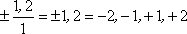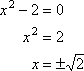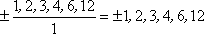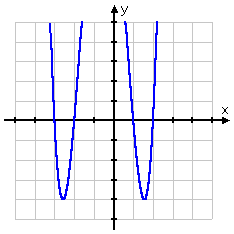Search

The Rational Roots Test: Introduction (page 1 of 2)The zero of a polynomial is an input value (usually an x-value) that returns a value of zero for the whole polynomial when you plug it into the polynomial. When a zero is a real (that is, not complex) number, it is also an x-intercept of the graph of the polynomial function. You will frequently (especially in calculus) want to know the location of the zeroes of a given polynomial function. You could plug numbers into the polynomial, willy-nilly, and hope for the best. But as you learned when you studied the Quadratic Formula, zeroes are often very messy numbers; randomly guessing is probably not the best plan of attack. So how does one go about trying to find zeroes?

The Rational Roots (or Rational Zeroes) Test is a handy way of obtaining a list of useful first guesses when you are trying to find the zeroes (roots) of a polynomial. Given a polynomial with integer (that is, positive and negative "whole-number") coefficients, the possible (or potential) zeroes are found by listing the factors of the constant (last) term over the factors of the leading coefficient, thus forming a list of fractions. This listing gives you a list of potential rational (fractional) roots to test -- hence the name of the Test.

Let me emphasize: The Rational Roots Test does not give you the zeroes. It does not say what the zeroes definitely will be. The Test only gives you a list of relatively easy and "nice" numbers to try in the polynomial. Most of these possible zeroes will turn out not actually to be zeroes!

You can see the sense of the Test's methodology by looking at a simple polynomial. Given the quadratic 12x2  7x  10, you can use the Quadratic Formula to find the zeroes, but you can also factor to get 12x2  7x  10 = (3x + 2)(4x  5). Setting the two factors equal to zero, you get two roots at x =  2/3 and x = 5/4. Note that the denominators "3" and "4" are factors of the leading coefficiant "12", and the numerators "2" and "5" are factors of the constant term "10". That is, the zeroes are fractions formed of factors of the constant term (10) over factors of the leading coefficient (12). Note also, however, that fractions such as 5/6 and  10/3 may also be formed this way (and thus be provided to you by the Test), but these other fractions are not in fact zeroes of this quadratic.

This relationship is always true: If a polynomial has rational roots, then those roots will be fractions of the form (plus-or-minus) (factor of the constant term) / (factor of the leading coefficient). However, not all fractions of this form are necessarily zeroes of the polynomial. Indeed, it may happen that none of the fractions so formed is actually a zero of the polynomial.

Note that I keep saying "potential" roots, "possible" zeroes, "if there are any such roots...". This is because the list of fractions generated by the Rational Roots Test is just a list of potential solutions. It need not be true that any of the fractions is actually a solution. There might not be any fractional roots! For example, given x2  2, the Rational Roots Tests gives the following possible rational zeroes:...so the zeroes aren't actually rational at all.

Always remember: The Rational Roots Test only gives a list of good first guesses; it does NOT give you "the" answers!

• Find all possible rational x-intercepts of x4 + 2x3  7x2  8x + 12.

The constant term is 12, with factors of 1, 2, 3, 4, 6, and 12. The leading coefficient in this case is just 1, which makes my work a lot simpler. The Rational Roots Test says that the possible zeroes are at: Copyright © Elizabeth Stapel 2002-2011 All Rights Reserved= 12, 6, 4, 3, 2, 1, 1, 2, 3, 4, 6, 12

 You can do a quick graph (especially if you have a graphing calculator), and see that, out of the above list, it would probably be good to start looking for zeroes by plugging the values x = 3, 2, 1, and 2 into the polynomial.Cite this article as: Stapel, Elizabeth. "The Rational Roots Test: Introduction." Purplemath. Available from     https://www.purplemath.com/modules/rtnlroot.htm. Accessed [Date] [Month] 2016

Study Skills Survey

Tutoring from Purplemath
Find a local math tutor